# Solutions

As mentioned within the session setup, load the following packages using the `library()` function. Additionally, as we will be using a data set with large numbers, set scipen to 999 using the option function.

``````  library(tidyverse)
library(RColorBrewer)

options(scipen = 999)``````

Furthermore, for the purpose of this session, we will be using data from the World Bank Open Data. In particular we will be using a collection of variables from 1999, these variables were selected to provide us plenty of room to explore!

It is included in your downloaded zip file from the accompanying Github Repo and can be loaded using the following code:

``WDB_1999 <- read_csv("../data/WDB_1999.csv")``

Section 1: ggplot2 vs plot

Exercise 1: Plotting birthrate against deathrate using both the `plot()` and `ggplot()` function, discuss which has more potential in displaying data clearly.

``````## Plotting using plot()

plot(data = WDB_1999,
birthrate ~ deathrate)``````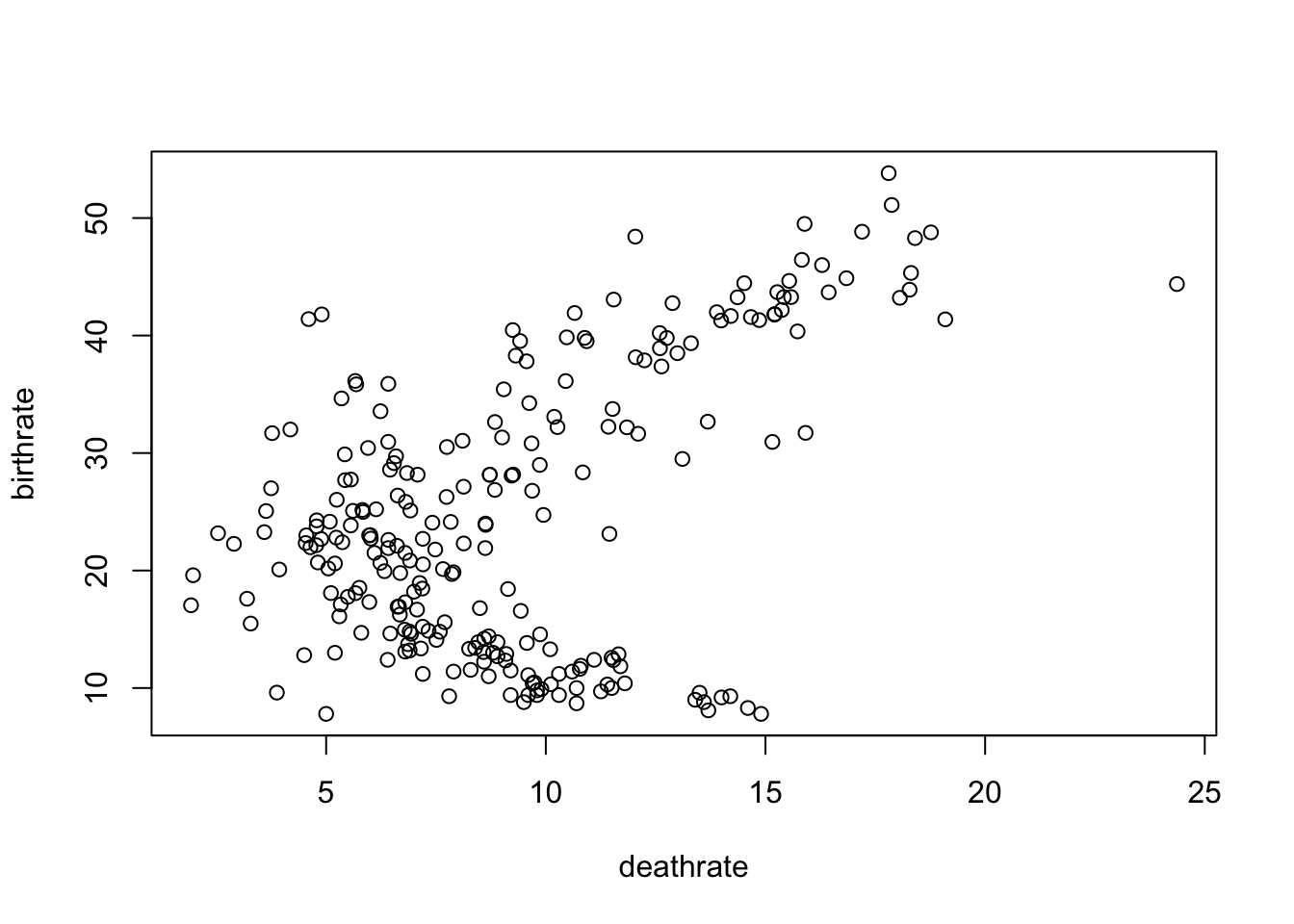``````## Plotting using ggplot()

ggplot(data = WDB_1999,
mapping = aes(
x = deathrate,
y = birthrate
)) +
geom_point()``````
``## Warning: Removed 17 rows containing missing values (geom_point).``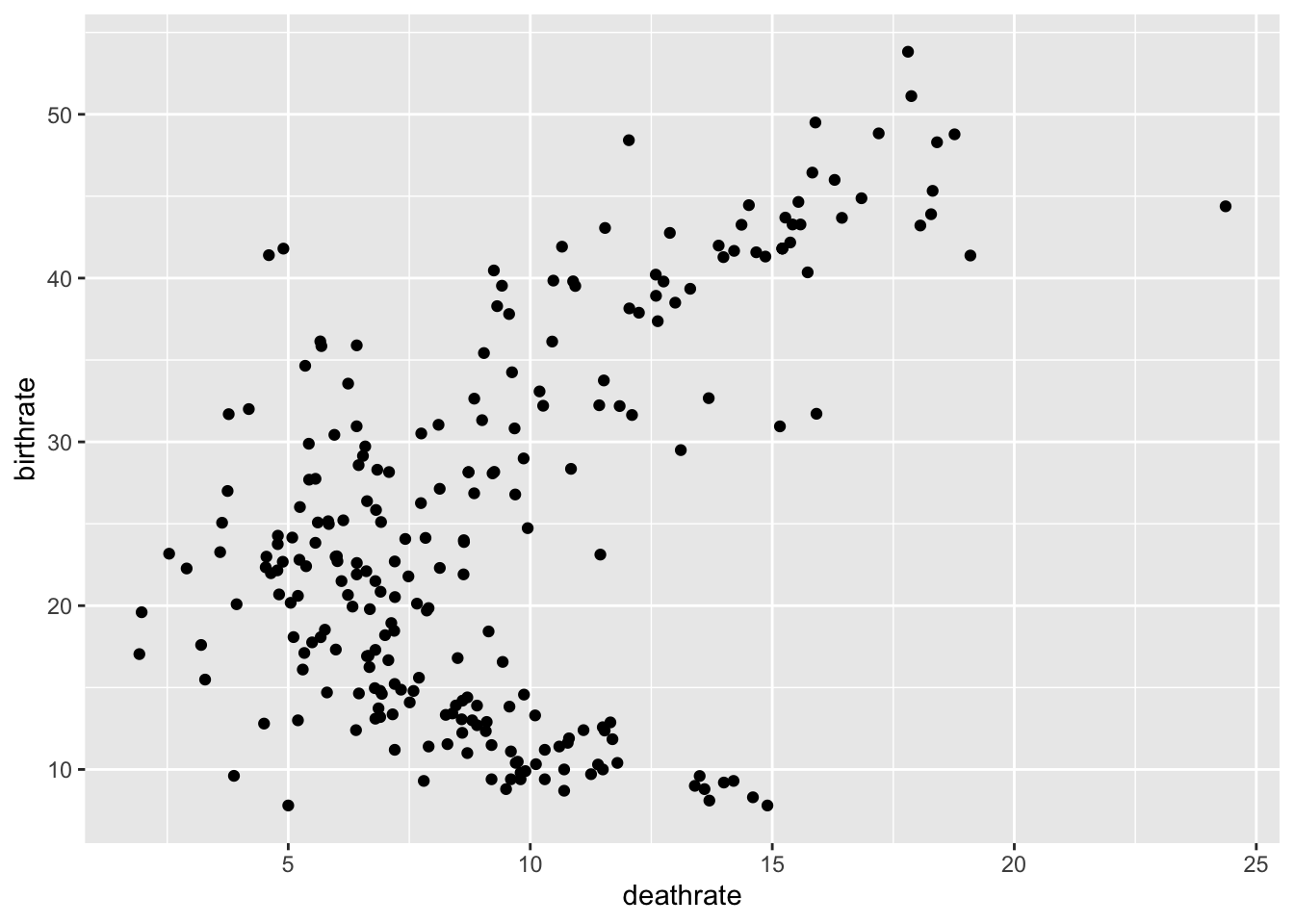Exercise 2: Expand the plot to group these points by Continent, which provides us with more information and is easier to achieve? Remember, you’ll need to recode WDB_1999\$Continent as a factor using the function:

``````## Plotting using plot()

WDB_1999\$Continent <- as.factor(WDB_1999\$Continent)

plot(data = WDB_1999,
birthrate ~ deathrate,
col = c("blue", "light blue", "red", "pink",
"green", "dark green", "black", "purple")[Continent])``````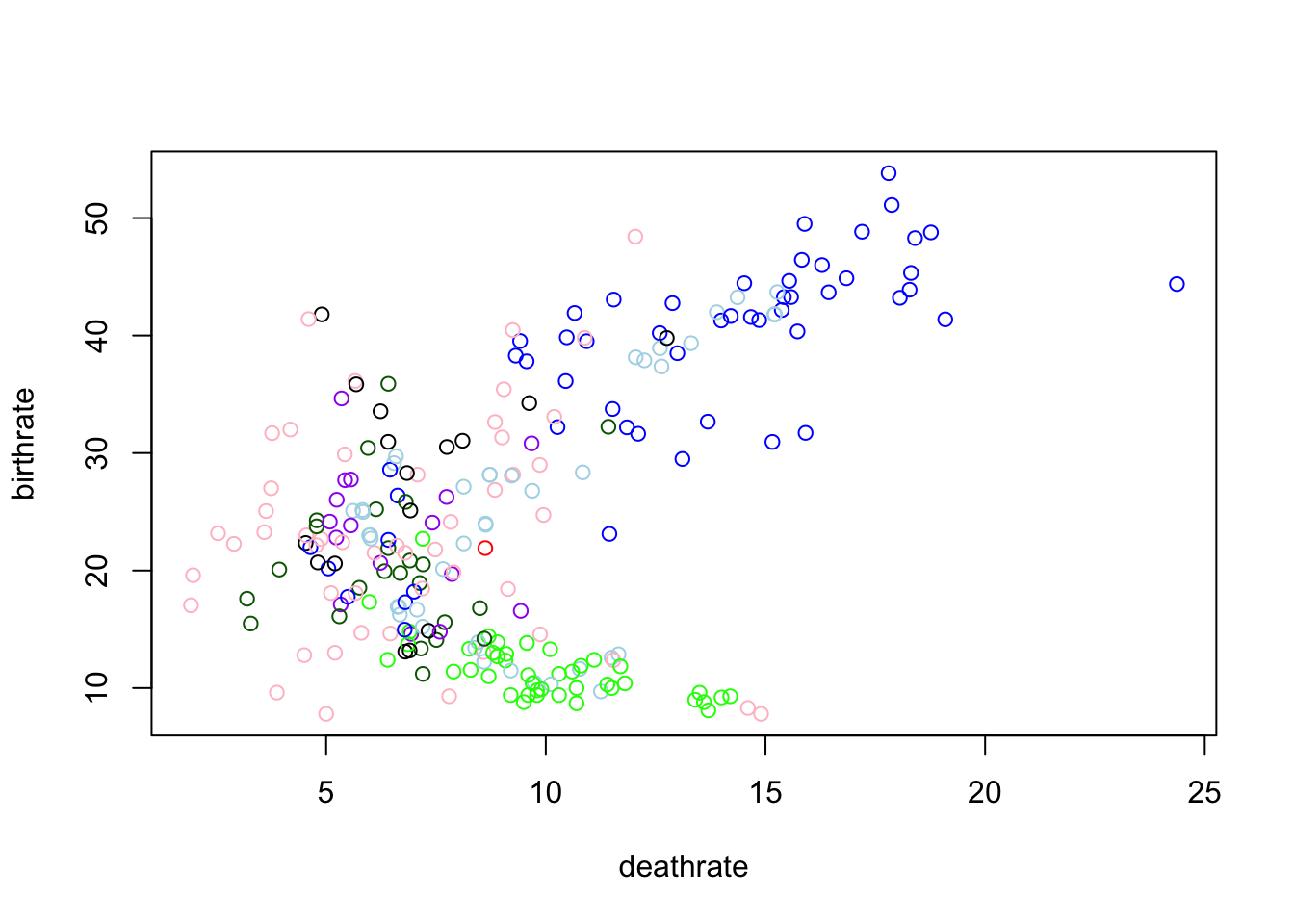``````## Plotting using ggplot()

ggplot(data = WDB_1999,
mapping = aes(
x = deathrate,
y = birthrate,
colour = Continent
)) +
geom_point()``````
``## Warning: Removed 17 rows containing missing values (geom_point).``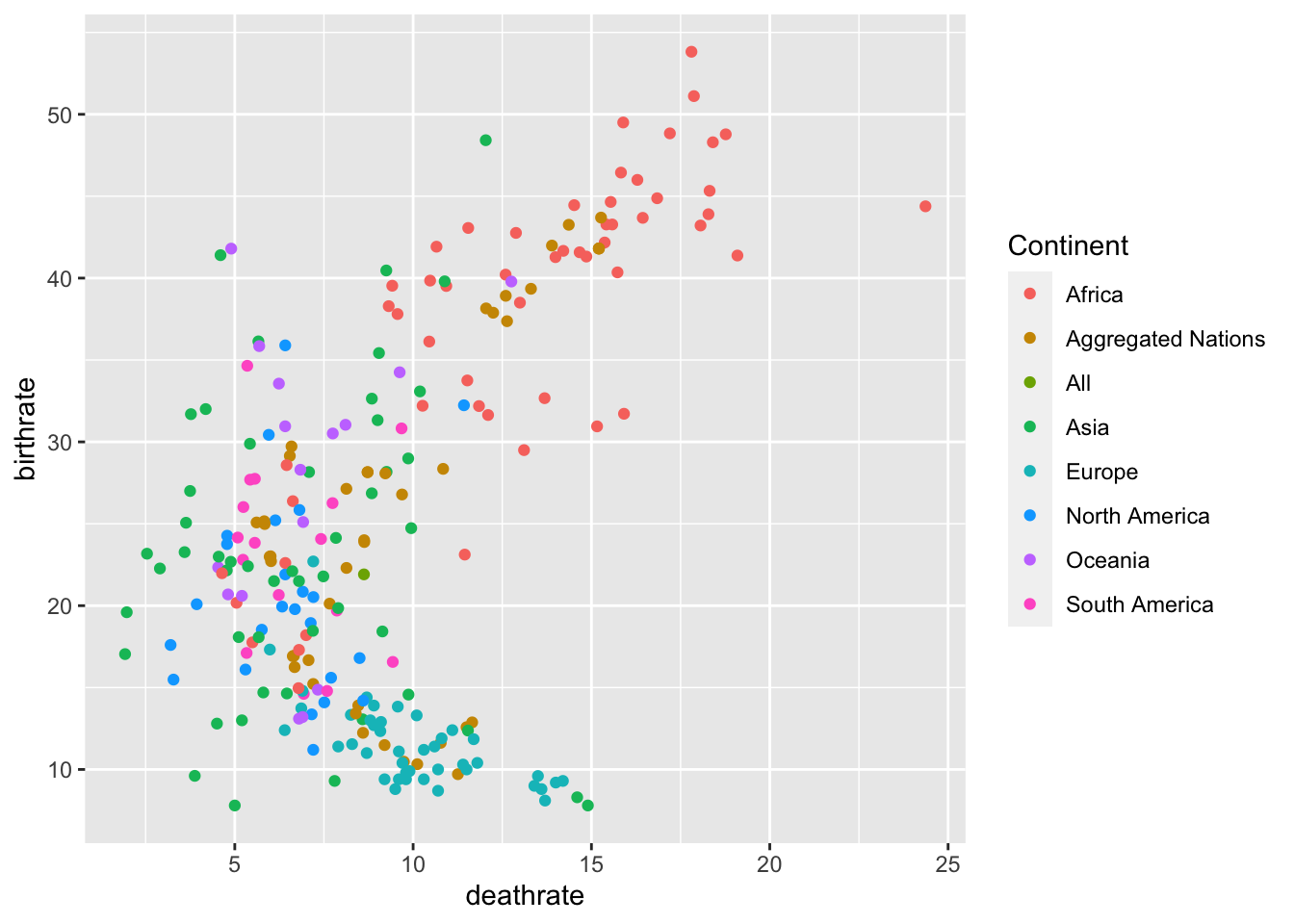Section 2: Scatter Plots in ggplot

Exercise 3: Change the size parameter to ed.years to see if there is a trend between amount of years in Education and the Birth and Death Rate, set the alpha parameter to 0.5 to clearly see the relationships.

``````  ggplot(data = WDB_1999,
mapping = aes(
x = birthrate,
y = deathrate,
colour = Continent,
size = ed.years
)) +
geom_point(alpha = 0.5)``````
``## Warning: Removed 69 rows containing missing values (geom_point).``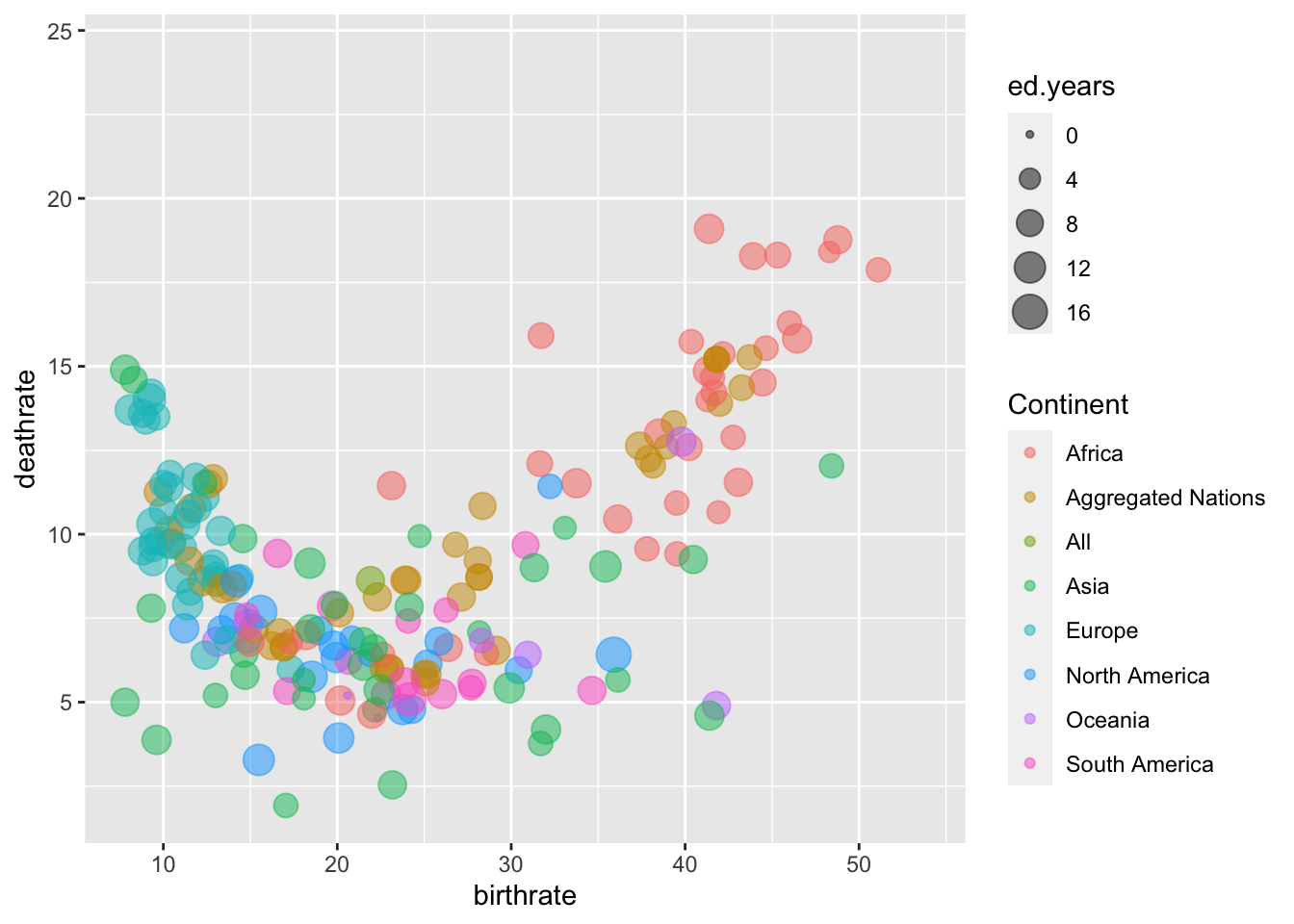Exercise 4: Change the Labels on the X and Y axis’ and provide a suitable title for the graph

``````  ggplot(data = WDB_1999,
mapping = aes(
x = birthrate,
y = deathrate,
colour = Continent,
size = ed.years
)) +
geom_point(alpha = 0.5) +
labs(x = "Birth Rate",
y = "Death Rate",
title = "Interaction between Birth and Death Rate.")``````
``## Warning: Removed 69 rows containing missing values (geom_point).``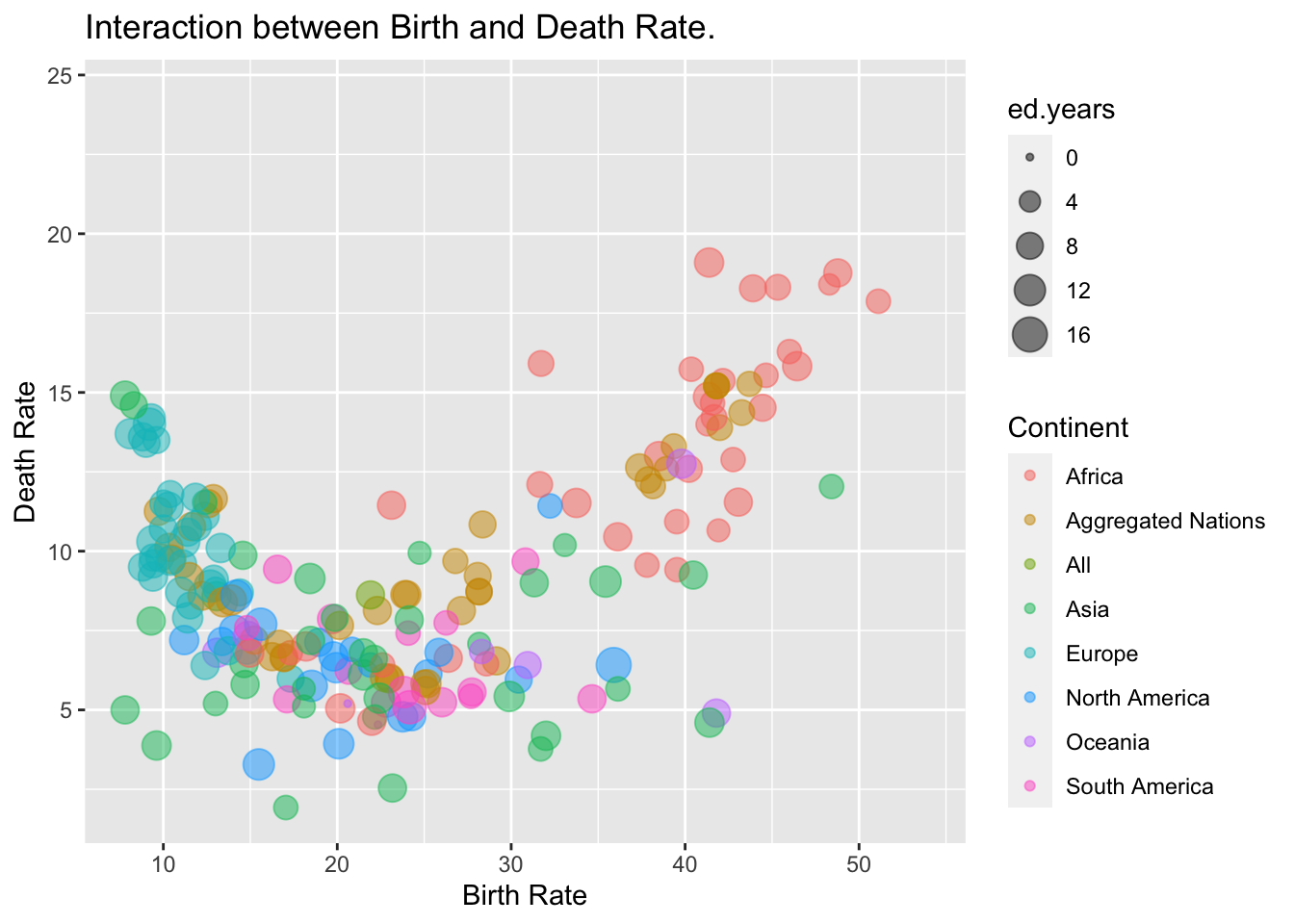Section 3: Bar Charts and Histograms

Exercise 5: Using the parameter `stat = "identity"` within the `geom_bar()` function, create a bar chart of `Continent` plotted against the mean `birthrate` or `deathrate`

``````  ggplot(data = WDB_1999) +
geom_bar(stat = "identity",
mapping = aes(x = Continent,
y = mean(birthrate, na.rm = TRUE))) ``````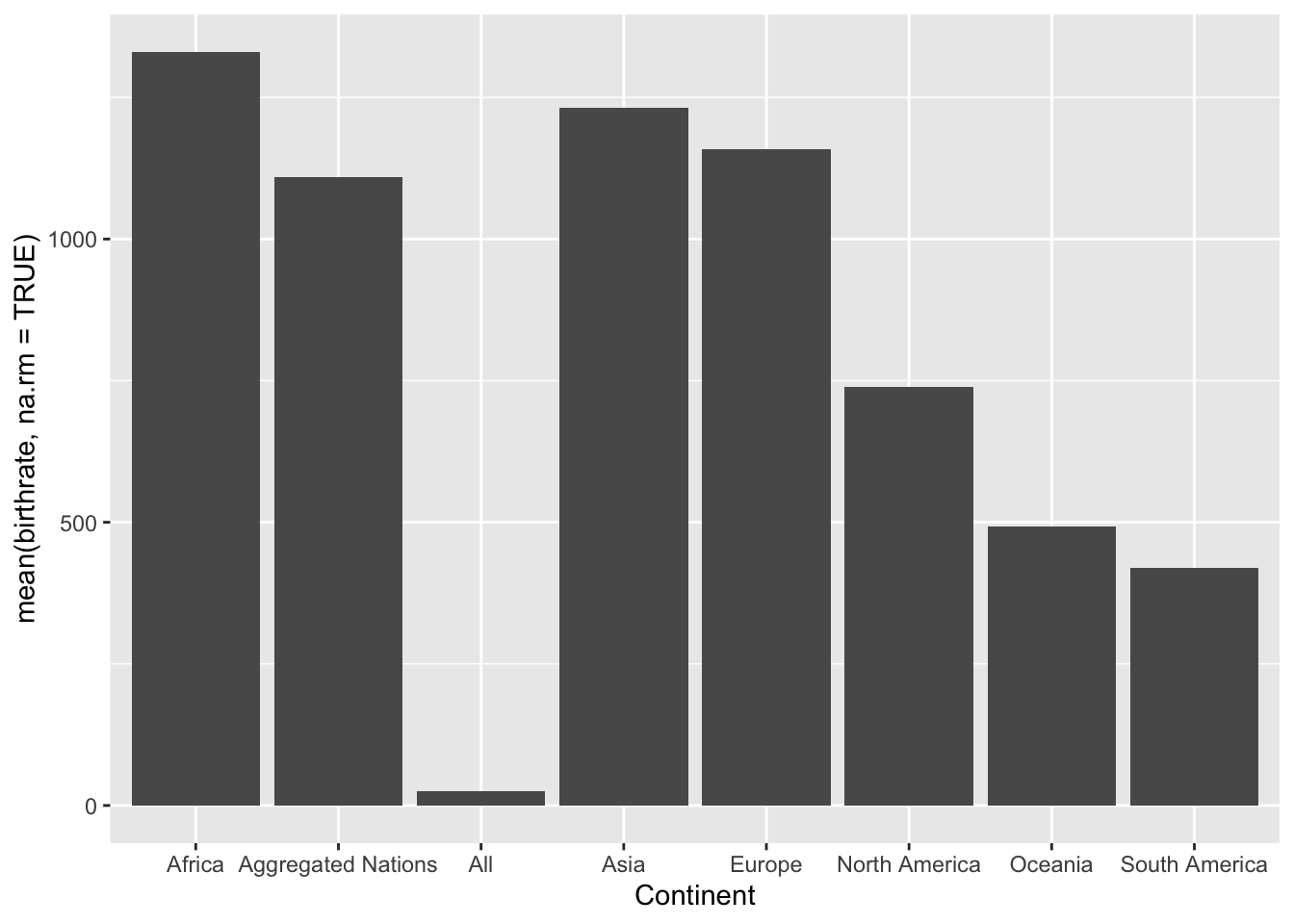``````  ggplot(data = WDB_1999) +
geom_bar(stat = "identity",
mapping = aes(x = Continent,
y = mean(deathrate, na.rm = TRUE))) ``````Exercise 6: Using the function `geom_histogram()` create a histogram of the birthrate and deathrate

``````  ggplot(data = WDB_1999) +
geom_histogram(mapping = aes(x = birthrate), colour = "blue", alpha = 0.5) +
geom_histogram(mapping = aes(x = deathrate), colour = "red", alpha = 0.5)``````
``## `stat_bin()` using `bins = 30`. Pick better value with `binwidth`.``
``## Warning: Removed 16 rows containing non-finite values (stat_bin).``
``## `stat_bin()` using `bins = 30`. Pick better value with `binwidth`.``
``## Warning: Removed 17 rows containing non-finite values (stat_bin).``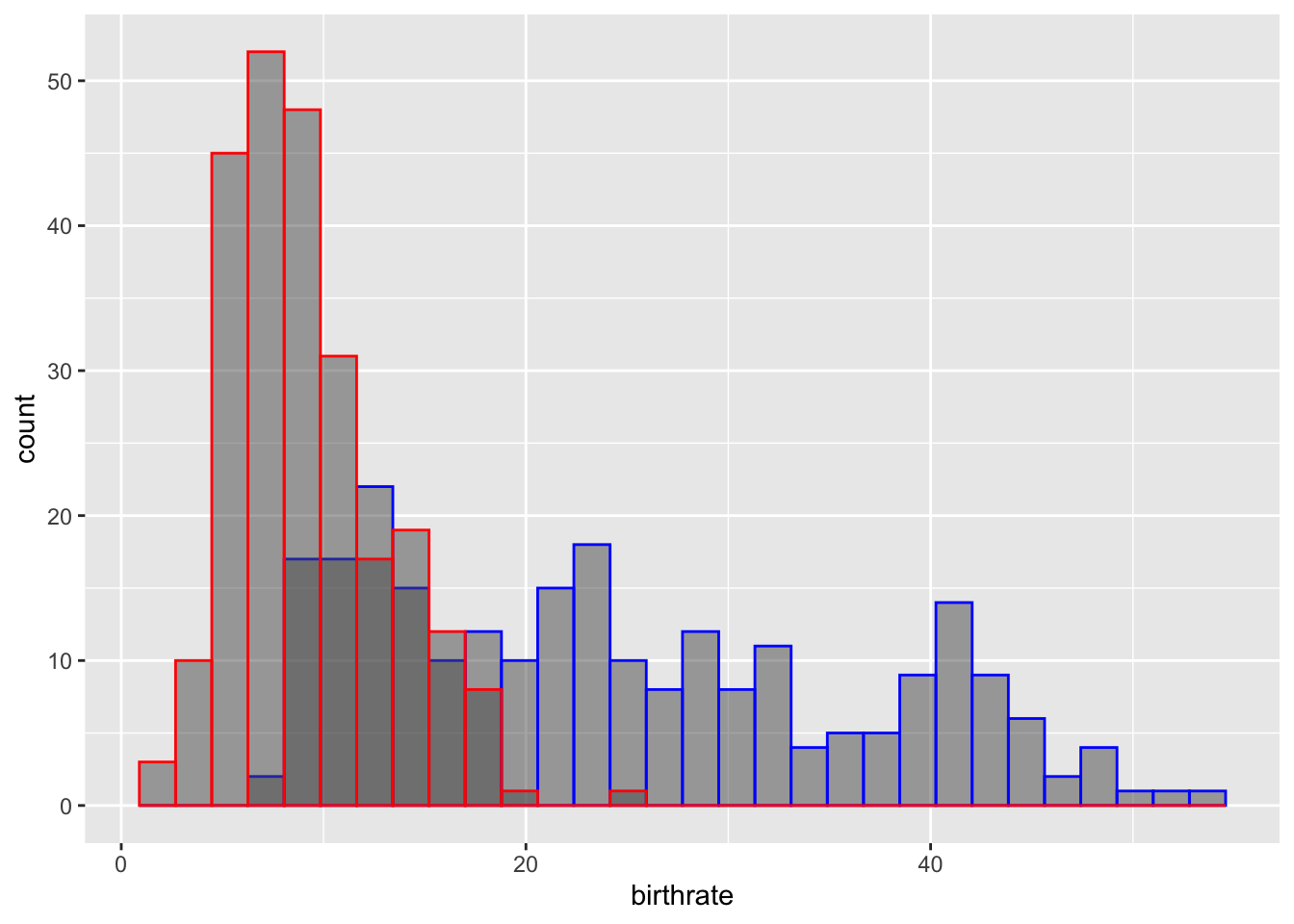Section 4: Adding density plots to Histograms

Exercise 7: Using the plot created in exercise 6, add the y-variable `..density..` and `binwidth = 1` to `geom_histogram()` in addition to adding `geom_density()` to add density lines to the Histogram

``````  ggplot(data = WDB_1999) +
geom_histogram(mapping = aes(x = birthrate, y = ..density..), binwidth = 1,
colour = "blue", alpha = 0.5) +
geom_histogram(mapping = aes(x = deathrate, y = ..density..), binwidth = 1,
colour = "red", alpha = 0.5) +
geom_density(mapping = aes(x = birthrate), colour = "blue", alpha = 0.5) +
geom_density(mapping = aes(x = deathrate), colour = "red", alpha = 0.5)``````
``## Warning: Removed 16 rows containing non-finite values (stat_bin).``
``## Warning: Removed 17 rows containing non-finite values (stat_bin).``
``## Warning: Removed 16 rows containing non-finite values (stat_density).``
``## Warning: Removed 17 rows containing non-finite values (stat_density).``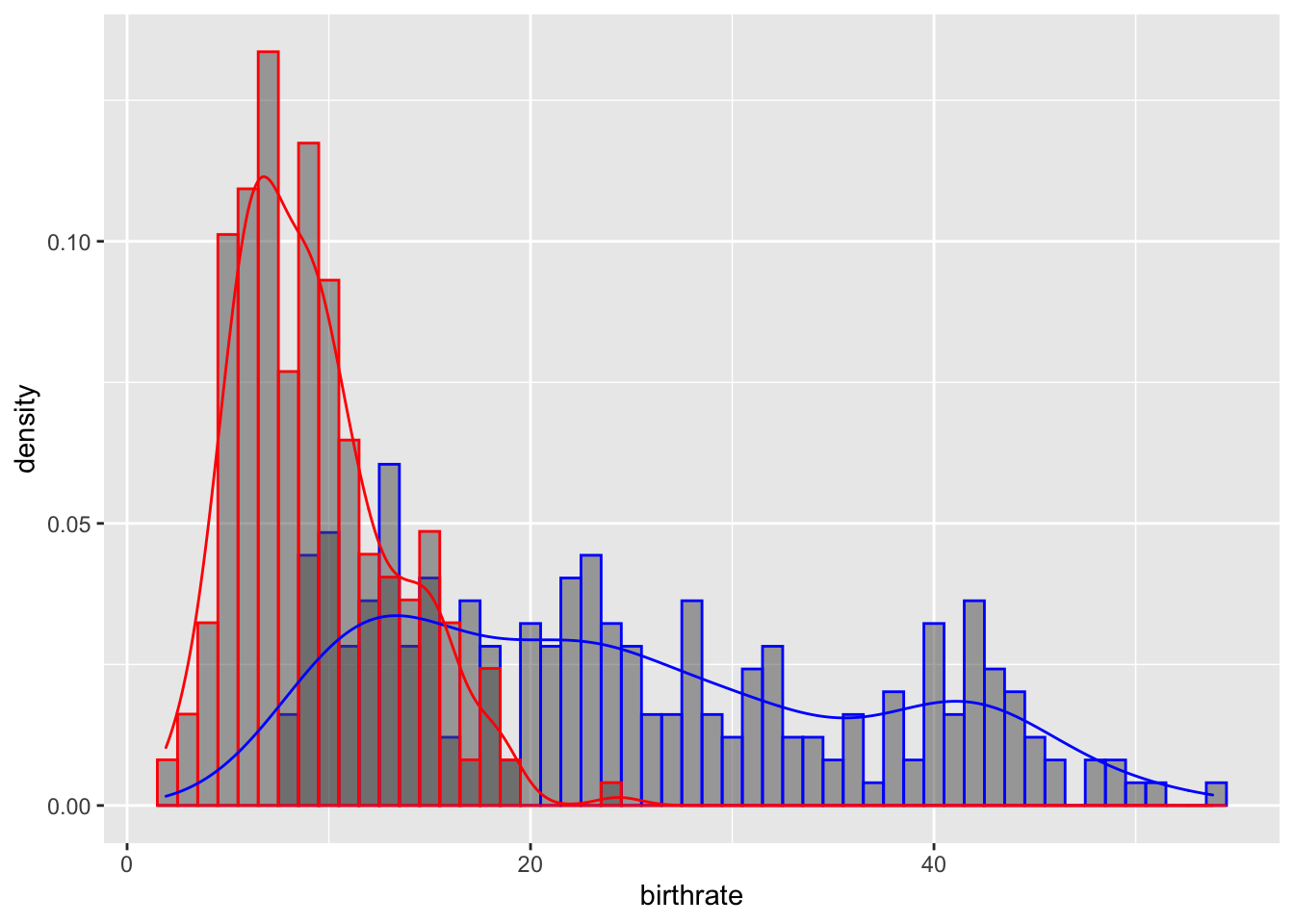Exercise 8: Add the parameter, `adjust = 2` in the density plot, to smooth this link and make it more easily interpretable

``````  ggplot(data = WDB_1999) +
geom_histogram(mapping = aes(x = birthrate, y = ..density..), binwidth = 1,
colour = "blue", alpha = 0.5) +
geom_histogram(mapping = aes(x = deathrate, y = ..density..), binwidth = 1,
colour = "red", alpha = 0.5) +
geom_density(mapping = aes(x = birthrate), colour = "blue", alpha = 0.5, adjust = 2) +
geom_density(mapping = aes(x = deathrate), colour = "red", alpha = 0.5, adjust = 2)``````

Section 5: Extra Useful Tips and Functions

Exercise 9: Use the `ggsave()` function to save your last plot

``````ggsave(filename = ??,
plot = last_plot())``````